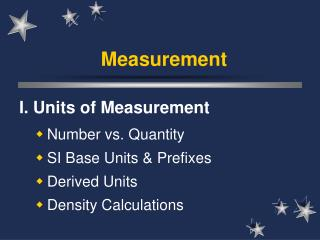DownloadDownload PresentationMeasurement

# Measurement

Download Presentation## Measurement

- - - - - - - - - - - - - - - - - - - - - - - - - - - E N D - - - - - - - - - - - - - - - - - - - - - - - - - - -
##### Presentation Transcript

1. Measurement I. Units of Measurement Number vs. Quantity SI Base Units & Prefixes Derived Units Density Calculations

2. A. Number vs. Quantity • Quantity - number + unit UNITS MATTER!!

3. B. SI Units Quantity Base Unit Symbol Length meter m Mass kilogram kg Time second s Temp kelvin K Current ampere A

4. mega- kilo- M k 103 106 deci- d 10-1 centi- c 10-2 milli- m 10-3 micro-  10-6 nano- n 10-9 pico- p 10-12 B. SI Units Prefix Symbol Factor

5. M V M D = D V C. Derived Units • Combination of base units. • Volume - length  length  length 1 cm3 = 1 mL 1 dm3 = 1 L • Density - mass per unit volume (g/cm3)

6. M D V D. Density • An object has a volume of 825 cm3 and a density of 13.6 g/cm3. Find its mass. GIVEN: V = 825 cm3 D = 13.6 g/cm3 M = ? WORK: M = DV M = (13.6 g/cm3)(825cm3) M = 11,220 g

7. WORK: V = M D V = 25 g 0.87 g/mL M D V D. Density 1) A liquid has a density of 0.87 g/mL. What volume is occupied by 25 g of the liquid? GIVEN: D = 0.87 g/mL V = ? M = 25 g V = 28.7 mL

8. WORK: D = M V D = 620 g 753 cm3 M D V D. Density 2) You have a sample with a mass of 620 g & a volume of 753 cm3. Find density. GIVEN: M = 620 g V = 753 cm3 D = ? D = 0.82 g/cm3